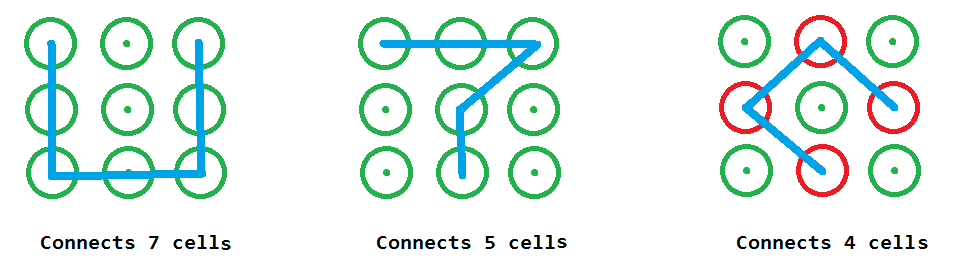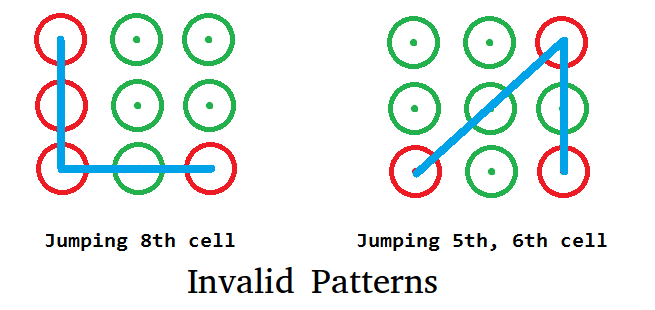# Number of ways to make mobile lock pattern

A mobile pattern is a grid of 3X3 cell, where drawing a specific pattern (connecting specific sequence of cells in order) will unlock the mobile. In this problem, the task is to calculate number of ways of making the lock pattern with number of connections in given range. In general terms, we are given a range as min and max, we need to tell how many patterns can be made which use at least min connection cell and at most max connection cell.

```Input  : min = 5, max = 7
Output : 106080
Below are some example patterns```

## Recommended: Please try your approach on {IDE} first, before moving on to the solution.

A simple solution is to do simple DFS by going through various connections from all starting points. We can optimize by seeing the symmetry between patterns, we can treat cell 1, 3, 7 and 9 as same. Similarly, we can treat 2, 4, 6 and 8 as same. Answer returned by a member of same group can be multiplied by 4 to get result by all members.
Next thing to note is that below patterns are not valid, because jumping some cell is not allowed as in below diagram cell 8 and cell 5, 6 are jumped.To take care of such invalid moves, a 2D jump array is taken in below code which stores possible jump cells in jump array. When we call recursively we impose an extra condition that if we are moving from one cell to another which involves some jumping cell, then this cell should be visited already to ignore invalid moves.
In below code we have made recursive calls from 1, 2 and 5 only because 1 will cover 3, 5 and 7 and 2 will cover 4, 6 and 8 due to symmetry.
See below code for better understanding :

## C++

 `//  C/C++ program to find number of ways to lock the mobile ` `// pattern ` `#include ` `using` `namespace` `std; ` `#define DOTS 10 ` ` `  `//  method to find total pattern starting from current cell ` `int` `totalPatternFromCur(``bool` `visit[DOTS], ``int` `jump[DOTS][DOTS], ` `                                          ``int` `cur, ``int` `toTouch) ` `{ ` `    ``if` `(toTouch <= 0) ` `    ``{ ` `        ``//  if last cell then return 1 way ` `        ``return` `(toTouch == 0)? 1 : 0; ` `    ``} ` ` `  `    ``int` `ways = 0; ` ` `  `    ``//  make this cell visited before going to next call ` `    ``visit[cur] = ``true``; ` ` `  `    ``for` `(``int` `i=1; i

## Java

 `// Java program to find number of ways  ` `// to lock the mobile pattern ` `class` `GFG  ` `{ ` `static` `int` `DOTS = ``10``; ` ` `  `// method to find total pattern starting from current cell ` `static` `int` `totalPatternFromCur(``boolean` `visit[], ``int` `jump[][], ` `                                       ``int` `cur, ``int` `toTouch) ` `{ ` `    ``if` `(toTouch <= ``0``) ` `    ``{ ` `        ``// if last cell then return 1 way ` `        ``return` `(toTouch == ``0``) ? ``1` `: ``0``; ` `    ``} ` ` `  `    ``int` `ways = ``0``; ` ` `  `    ``// make this cell visited before  ` `    ``// going to next call ` `    ``visit[cur] = ``true``; ` ` `  `    ``for` `(``int` `i = ``1``; i < DOTS; i++) ` `    ``{ ` `         `  `    ``/* if this cell is not visit AND ` `        ``either i and cur are adjacent (then jump[i][cur] = 0) ` `        ``or between cell must be visit already ( ` `        ``then visit[jump[i][cur]] = 1) */` `    ``if` `(!visit[i] && (jump[i][cur] == ``0` `||  ` `         ``visit[jump[i][cur]])) ` `        ``ways += totalPatternFromCur(visit, jump,  ` `                                    ``i, toTouch - ``1``); ` `    ``} ` ` `  `    ``// make this cell not visited ` `    ``// after returning from call ` `    ``visit[cur] = ``false``; ` ` `  `    ``return` `ways; ` `} ` ` `  `// method returns number of pattern with  ` `// minimum m connection and maximum n connection ` `static` `int` `waysOfConnection(``int` `m, ``int` `n) ` `{ ` `    ``int` `[][]jump = ``new` `int``[DOTS][DOTS]; ` ` `  `    ``// 2 lies between 1 and 3 ` `    ``jump[``1``][``3``] = jump[``3``][``1``] = ``2``; ` ` `  `    ``// 8 lies between 7 and 9 ` `    ``jump[``7``][``9``] = jump[``9``][``7``] = ``8``; ` ` `  `    ``// 4 lies between 1 and 7 ` `    ``jump[``1``][``7``] = jump[``7``][``1``] = ``4``; ` ` `  `    ``// 6 lies between 3 and 9 ` `    ``jump[``3``][``9``] = jump[``9``][``3``] = ``6``; ` ` `  `    ``// 5 lies between 1, 9 2, 8 3, 7 and 4, 6 ` `    ``jump[``1``][``9``] = jump[``9``][``1``] = jump[``2``][``8``] =  ` `                 ``jump[``8``][``2``] = jump[``3``][``7``] =  ` `                 ``jump[``7``][``3``] = jump[``4``][``6``] =  ` `                 ``jump[``6``][``4``] = ``5``; ` ` `  `    ``boolean` `[]visit = ``new` `boolean``[DOTS]; ` `    ``int` `ways = ``0``; ` `    ``for` `(``int` `i = m; i <= n; i++) ` `    ``{ ` `        ``// 1, 3, 7, 9 are symmetric so multiplying by 4 ` `        ``ways += ``4` `* totalPatternFromCur(visit,  ` `                                        ``jump, ``1``, i - ``1``); ` ` `  `        ``// 2, 4, 6, 8 are symmetric so multiplying by 4 ` `        ``ways += ``4` `* totalPatternFromCur(visit, ` `                                        ``jump, ``2``, i - ``1``); ` ` `  `        ``ways += totalPatternFromCur(visit,  ` `                                    ``jump, ``5``, i - ``1``); ` `    ``} ` `    ``return` `ways; ` `} ` ` `  `// Driver Code ` `public` `static` `void` `main(String[] args)  ` `{ ` `    ``int` `minConnect = ``4``; ` `    ``int` `maxConnect = ``6``; ` ` `  `    ``System.out.println(waysOfConnection(minConnect, ` `                                        ``maxConnect)); ` `} ` `} ` ` `  `// This code is contributed by PrinciRaj1992 `

## C#

 `// C# program to find number of ways  ` `// to lock the mobile pattern ` `using` `System; ` `     `  `class` `GFG  ` `{ ` `static` `int` `DOTS = 10; ` ` `  `// method to find total pattern starting from current cell ` `static` `int` `totalPatternFromCur(Boolean []visit, ``int` `[,]jump, ` `                                       ``int` `cur, ``int` `toTouch) ` `{ ` `    ``if` `(toTouch <= 0) ` `    ``{ ` `        ``// if last cell then return 1 way ` `        ``return` `(toTouch == 0) ? 1 : 0; ` `    ``} ` ` `  `    ``int` `ways = 0; ` ` `  `    ``// make this cell visited before  ` `    ``// going to next call ` `    ``visit[cur] = ``true``; ` ` `  `    ``for` `(``int` `i = 1; i < DOTS; i++) ` `    ``{ ` `         `  `    ``/* if this cell is not visit AND ` `        ``either i and cur are adjacent (then jump[i,cur] = 0) ` `        ``or between cell must be visit already ( ` `        ``then visit[jump[i,cur]] = 1) */` `    ``if` `(!visit[i] && (jump[i, cur] == 0 ||  ` `        ``visit[jump[i, cur]])) ` `        ``ways += totalPatternFromCur(visit, jump,  ` `                                    ``i, toTouch - 1); ` `    ``} ` ` `  `    ``// make this cell not visited ` `    ``// after returning from call ` `    ``visit[cur] = ``false``; ` ` `  `    ``return` `ways; ` `} ` ` `  `// method returns number of pattern with  ` `// minimum m connection and maximum n connection ` `static` `int` `waysOfConnection(``int` `m, ``int` `n) ` `{ ` `    ``int` `[,]jump = ``new` `int``[DOTS, DOTS]; ` `  `  `    ``// 2 lies between 1 and 3 ` `    ``jump[1, 3] = jump[3, 1] = 2; ` ` `  `    ``// 8 lies between 7 and 9 ` `    ``jump[7, 9] = jump[9, 7] = 8; ` ` `  `    ``// 4 lies between 1 and 7 ` `    ``jump[1, 7] = jump[7, 1] = 4; ` ` `  `    ``// 6 lies between 3 and 9 ` `    ``jump[3, 9] = jump[9, 3] = 6; ` ` `  `    ``// 5 lies between 1, 9 2, 8 3, 7 and 4, 6 ` `    ``jump[1, 9] = jump[9, 1] = jump[2, 8] =  ` `                 ``jump[8, 2] = jump[3, 7] =  ` `                 ``jump[7, 3] = jump[4, 6] =  ` `                 ``jump[6, 4] = 5; ` ` `  `    ``Boolean []visit = ``new` `Boolean[DOTS]; ` `    ``int` `ways = 0; ` `    ``for` `(``int` `i = m; i <= n; i++) ` `    ``{ ` `        ``// 1, 3, 7, 9 are symmetric so multiplying by 4 ` `        ``ways += 4 * totalPatternFromCur(visit,  ` `                                        ``jump, 1, i - 1); ` ` `  `        ``// 2, 4, 6, 8 are symmetric so multiplying by 4 ` `        ``ways += 4 * totalPatternFromCur(visit, ` `                                        ``jump, 2, i - 1); ` ` `  `        ``ways += totalPatternFromCur(visit,  ` `                                    ``jump, 5, i - 1); ` `    ``} ` `    ``return` `ways; ` `} ` ` `  `// Driver Code ` `public` `static` `void` `Main(String[] args)  ` `{ ` `    ``int` `minConnect = 4; ` `    ``int` `maxConnect = 6; ` ` `  `    ``Console.WriteLine(waysOfConnection(minConnect, ` `                                       ``maxConnect)); ` `} ` `} ` ` `  `// This code is contributed by 29AjayKumar `

Output:

```34792
```

This article is contributed by Utkarsh Trivedi. If you like GeeksforGeeks and would like to contribute, you can also write an article using contribute.geeksforgeeks.org or mail your article to contribute@geeksforgeeks.org. See your article appearing on the GeeksforGeeks main page and help other Geeks.

My Personal Notes arrow_drop_up

Improved By : princiraj1992, 29AjayKumar

Article Tags :
Practice Tags :

Be the First to upvote.

Please write to us at contribute@geeksforgeeks.org to report any issue with the above content.